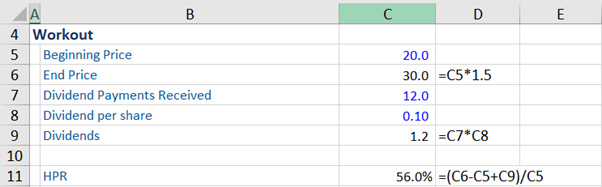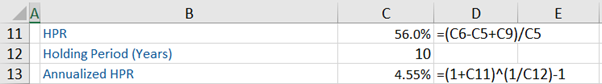## What are the “Components of Return?”

Investment returns can be measured in several ways, as returns are multi-dimensional, and the most comprehensive measure of return is known as total return. The components of return or total return refers to or incorporates two primary sources of return that an investor earns from an investment or an investment portfolio.

These two components of return are income, which includes interest payments on fixed-income investments, dividends from stocks, or distributions that an investor receives, and capital appreciation (i.e. the increase in the value of an asset or security, which represents the change in the market price of the same) realized over a given period.

## Key Learning Points

• In many cases, total return is also referred to as holding period return, which again includes the above-mentioned (two) components of return. The holding period can be for a day, one month, 1 year or 5 years etc; and
• If the market price of an asset or security falls, instead of increasing, it will result in a capital loss, which in turn will adversely impact the holding or total return on investment.

## Holding Period Return (HPR) and Components of Return

Total return is also referred to as holding period return in many cases. For investments, holding period return refers to the total return earned from an investment or an investment portfolio over the period for which the asset or the portfolio was held by that investor, which could be 1 day, 1 month, 1 year, 5 years etc. and again it incorporates the two components of return (i.e. both income and capital appreciation (or loss)) in the investment.

To compute the holding period return (HPR), which is a measure of an investment’s performance, the formula is:

HPR = (Market price (end period) – Market Price (beginning period) + Income)/Market Price (beginning period)

Income refers to the income an investor received along the way, in the form of interest or dividends (these are the periodic cash flows that an investor receives from the investment). The holding period refers to the period between the purchase and sale of a security.

The result of the above formula is either a gain (%) or loss (%) on the investment. For example, if the holding period return on a security is 25%, it means that the security increased by 25% of its original value due to the components of return that comprise of capital appreciation (due to increase in the market price of the security) and income (in the form of dividends (in the case of stocks), or coupons (in the case of bonds)) received over a given period of time. Investments that do not generate a stream of interest or dividend income, will only possess the capital appreciation component.

Having stated the above, if the market price of the security falls, instead of increasing, the investor will face a capital loss.

It is worth noting that the above HPR formula is not an annualized number. However, sometimes, one does see it as an annualized figure.

## Components of Return, Example

Given below are examples of the total return calculation. Assume that an investor paid \$20 per share (beginning price) for 200 shares in company XYZ. Further, assume that the current share price has increased by 50% to \$30. The investor has also received 12 dividend payments, each amounting to \$0.10 per share. Now, if this investor decides to sell all his or her shares at the current price of \$30, the holding period return (HPR) is 56%.Next, given below is the computation of the annualized HPR, assuming that this investor bought these shares 10 years ago.In another example, given below, assume that the 10-year annualized total return for the S&P 500 is 15%. Further, assume that during this period, the price of a stock increased from \$797.87 to \$2,703.40.

Given this information, we see the components of return are the return from capital appreciation is 239% and return from dividends is 2% during this period. Next, we annualize the return from capital appreciation, which as per the calculation is 13%.

To obtain the total return, we add return from capital appreciation (annualized) and return from dividends to obtain the total return of 15%.